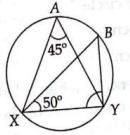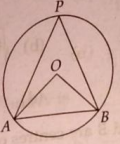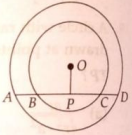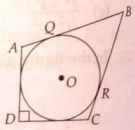Friday, October 22, 2021
Home > CBSE Class 10 > Extra MCQ Questions for Class 10 Maths Chapter 10 Tangent to Circles

# Extra MCQ Questions for Class 10 Maths Chapter 10 Tangent to CirclesHi students, Welcome to AMBiPi (Amans Maths Blogs). In this article, you will get Extra MCQ Questions for Class 10 Maths Chapter 10 Tangent to Circles. You can download this PDF and save it in your mobile device or laptop etc.

Extra MCQ Questions for Class 10 Maths Circles Question No 31:

Two circles cut each other at points P and Q. Centres of two circles are respectively A and B and PA is perpendicular to PB. If AB intersects segment PQ at R and ratio of the two circles are respectively 16 : 9, then find the ratio of AR : BR.

Option A : 4 : 3

Option B : 16 : 9

Option C : 256 : 81

Option D : 2 : √3

Option B : 16 : 9

Extra MCQ Questions for Class 10 Maths Circles Question No 32:

In the figure below, find the length of side AC.Option A : 20

Option B : 22

Option C : 21

Option D : 18

Option B : 22

Extra MCQ Questions for Class 10 Maths Circles Question No 33:

PQ is a line segment of 12 cm whose midpoint is R. Taking PR, RQ and PQ as diameters semicircles are drawn at the same side of PQ. Find the area of the circle that touches all the three circles.

Option A : 2π sq. m

Option B : 4π sq. m

Option C : 6π sq. m

Option D : 9π/4 sq. m

Option B : 4π sq. m

Extra MCQ Questions for Class 10 Maths Circles Question No 34:

The difference of the lengths of the parallel sides of a trapezium inscribed in a circle is 6 cm. If the distance between parallel sides is 4 cm then difference in lengths of its non parallel sides is

Option A : 10 cm

Option B : 5 cm

Option C : 0 cm

Option D : None of these

Option C : 0 cm

Extra MCQ Questions for Class 10 Maths Circles Question No 35:

In the figure below, find the angle BYX.Option A : 85o

Option B : 50o

Option C : 45o

Option D : 90o

Option A : 85 degree

Extra MCQ Questions for Class 10 Maths Circles Question No 36:

In the figure below, OA is equal to chord AB. Find the value of ∠APB.Option A : 30

Option B : 60

Option C : 15

Option D : 45

Option A : 30

Extra MCQ Questions for Class 10 Maths Circles Question No 37:

In the figure below, T is a point outside the circle such that OT = 13 and radius of the circle is 5. If two tangents TP and TQ are drawn from T, find the length of AB.Option A : 19/3 cm

Option B : 20/3 cm

Option C : 40/13 cm

Option D : 22/3 cm

Option B : 20/3 cm

Extra MCQ Questions for Class 10 Maths Circles Question No 38:

In the figure below, AD is a straight line. OP is perpendicular to AD and O is the center of the both circles. If OA = 20 cm, OB = 15 cm and OP = 12 cm, then find the length of AB.Option A : 7 cm

Option B : 8 cm

Option C : 10 cm

Option D : 12 cm

Option A : 7 cm

Extra MCQ Questions for Class 10 Maths Circles Question No 39:

In the figure below, a circle is inscribed in the quadrilateral ABCD. If BC = 38 cm, QB = 27 cm and DC = 25 cm, if AD is perpendicular to DC, then find the radius the circle?Option A : 11 cm

Option B : 14 cm

Option C : 15 cm

Option D : 16 cm

Option B : 14 cm

Extra MCQ Questions for Class 10 Maths Circles Question No 40:

In the figure below, find the angle CBA.Option A : 30

Option B : 45

Option C : 50

Option D : 60